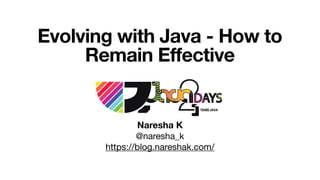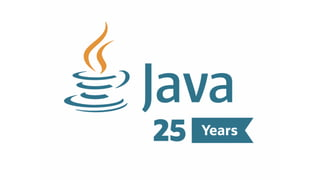Successfully reported this slideshow.×
1 of 74

Evolving with Java - How to Remain Effective

0

Share

Slides from my Java2Days 2020 talk - "Evolving with Java - How to Remain Effective".

Developers find themselves in need to continually update themselves with the rapidly changing technologies to remain relevant and deliver value. However, by keeping a few things in mind and with certain practices, this can be a pleasant experience. In this presentation, I share my experiences learning and evolving with Java in the last 15+ years. The ideas presented are generic enough to be applicable for people using any technology stack. However, the code examples are in Java/ JVM languages.

We start by understanding the importance of gradual improvement. To keep motivated for continuous improvement, in my experience, responsiveness is a vital element. I share my experience of how to increase your responsiveness. To be able to change/ experiment continuously in our code, we need to ensure that we don't break anything. We explore the necessary techniques to achieve safety. Often we mistakenly consider lack of familiarity as complexity. We explore options to come out of this confusion. We then touch upon the impact of learning paradigms and multiple languages available on the JVM. Finally, we touch upon another important aspect of continuous improvement that is unlearning. We conclude the session by summarising the principles.

See all

See all

Evolving with Java - How to Remain Effective

1. 1. Evolving with Java - How to Remain Effective Naresha K @naresha_k https://blog.nareshak.com/
2. 2. About me Developer, Architect & Tech Excellence Coach Founder & Organiser Bangalore Groovy User Group
4. 4. Time to Market | Speed of Delivery
5. 5. Economy
6. 6. public static void main(String[] args) { List<Integer> numbers = List.of(1, 2, 3, 4, 5, 6); List<Integer> result = numbers.stream() .map(ParallelStreams::computeHeavyArithmetic) .collect(Collectors.toList()); System.out.println(result); } public static void main(String[] args) { List<Integer> numbers = List.of(1, 2, 3, 4, 5, 6); List<Integer> result = numbers.parallelStream() .map(ParallelStreams::computeHeavyArithmetic) .collect(Collectors.toList()); System.out.println(result); }
7. 7. public static void main(String[] args) { List<Integer> numbers = List.of(1, 2, 3, 4, 5, 6); List<Integer> result = numbers.stream() .map(ParallelStreams::computeHeavyArithmetic) .collect(Collectors.toList()); System.out.println(result); } public static void main(String[] args) { List<Integer> numbers = List.of(1, 2, 3, 4, 5, 6); List<Integer> result = numbers.parallelStream() .map(ParallelStreams::computeHeavyArithmetic) .collect(Collectors.toList()); System.out.println(result); }
8. 8. private static Logger LOGGER = LoggerFactory .getLogger(MyClass.class);
9. 9. All models are wrong, but some are useful. https://en.wikipedia.org/wiki/All_models_are_wrong George Box
10. 10. Pain!
11. 11. Suffering Pain!
12. 12. I have not experienced the pain, yet.
13. 13. public static String concatWithPlus(String[] values) { String result = ""; for (int i = 0; i < values.length; i++) { result += values[i]; } return result; }
14. 14. public static String concatWithStringBuffer(String[] values) { StringBuffer buffer = new StringBuffer(); for (int i = 0; i < values.length; i++) { buffer.append(values[i]); } return buffer.toString(); }
15. 15. Java 1.4
16. 16. Java 5
17. 17. Maintainability?
18. 18. ‘+’ vs StringBuffer API Contract Concatenation Thread Safety Level of Abstraction Concatenation
19. 19. Favour higher level of abstraction
20. 20. Premature optimisation is the root of all evil ~ Donald Knuth
21. 21. Rule 1: Don’t Rule 2: Don’t, yet Rule 3: Profile before optimising http://wiki.c2.com/?RulesOfOptimization
22. 22. YAGNI
23. 23. Make it work Make it better
24. 24. Make it work Make it Right Make it Fast http://wiki.c2.com/?MakeItWorkMakeItRightMakeItFast
25. 25. Java 11
26. 26. Red Green Refactor TDD
27. 27. /** * This method returns orders of customer * @param customer Customer whose orders to be fetched * @return List containing Order objects of the * specified Customer */ public List getOrdersOfCustomer(Customer customer);
28. 28. public List<Order> getOrdersOfCustomer(Customer customer); public List<OrderSummary> getOrdersOfCustomer(Customer customer);
29. 29. Self Documenting Code
30. 30. public static void main(String[] args) { List numbers = Arrays.asList(1, 2, 3, 4, 5); Iterator iterator = numbers.iterator(); while (iterator.hasNext()) { Number number = (Number) iterator.next(); System.out.println(number); } } Java 1.4
31. 31. public static void main(String[] args) { List numbers = Arrays.asList(1, 2, 3, 4, 5); Iterator iterator = numbers.iterator(); while (iterator.hasNext()) { Number number = (Number) iterator.next(); System.out.println(number); } } Java 1.4 List<Number> numbers = Arrays.asList(1, 2, 3, 4, 5); for(Number number : numbers) { System.out.println(number); } Java 5
32. 32. Syntactic Sugar
33. 33. Minimise Moving Parts
34. 34. List<Integer> numbers = List.of(1, 2, 3, 4, 5, 6); int sumOfSquaresOfEvenNumbers = 0; for (Integer number : numbers) { if(number % 2 == 0) { sumOfSquaresOfEvenNumbers += number * number; } } System.out.println(sumOfSquaresOfEvenNumbers);
35. 35. List<Integer> numbers = List.of(1, 2, 3, 4, 5, 6); int sumOfSquaresOfEvenNumbers = 0; for (Integer number : numbers) { if(number % 2 == 0) { sumOfSquaresOfEvenNumbers += number * number; } } System.out.println(sumOfSquaresOfEvenNumbers); Predicate<Integer> isEven = (number) -> number % 2 == 0; Function<Integer, Integer> square = (number) -> number * number; List<Integer> numbers = List.of(1, 2, 3, 4, 5, 6); Integer sum = numbers.stream() .filter(isEven) .map(square) .collect(Collectors. summingInt(Integer::intValue)); System.out.println(sum); Java 8
36. 36. Favour Declarative Code
38. 38. Predicate<Integer> isEven = (number) -> number % 2 == 0; Function<Integer, Integer> square = (number) -> number * number; List<Integer> numbers = List.of(1, 2, 3, 4, 5, 6); Integer result = numbers.stream() .filter(isEven) .map(square) .findFirst().orElse(-1); System.out.println(result); Java 8
39. 39. Predicate<Integer> isEven = (number) -> number % 2 == 0; Function<Integer, Integer> square = (number) -> number * number; List<Integer> numbers = List.of(1, 2, 3, 4, 5, 6); Integer result = numbers.stream() .filter(isEven) .map(square) .findFirst().orElse(-1); System.out.println(result); List<Integer> numbers = List.of(1, 2, 3, 4, 5, 6); int suqareOfFirstEvenNumber = -1; for (Integer number : numbers) { if(number % 2 == 0) { suqareOfFirstEvenNumber += number * number; break; } } System.out.println(suqareOfFirstEvenNumber); Java 8
40. 40. What you see is not what you get
41. 41. Lazy Evaluation
42. 42. Understand one level below the abstraction you deal with
43. 43. Introspector.java
44. 44. Food pizza = new Pizza(); Person friend = new Person(“Raj"); friend.getMouth().setFood(pizza);
45. 45. Food pizza = new Pizza(); Person friend = new Person(“Raj"); friend.getMouth().setFood(pizza); Person friend = new Person(“Raj"); Edible food = new Pizza(); friend.offer(food);
46. 46. Encapsulation
47. 47. Use checked exceptions Judiciously
48. 48. 55 Most people talk about Java the language, and this may sound odd coming from me, but I could hardly care less. At the core of the Java ecosystem is the JVM. - James Gosling, Creator of the Java Programming Language(2011, TheServerSide)